Successfully reported this slideshow.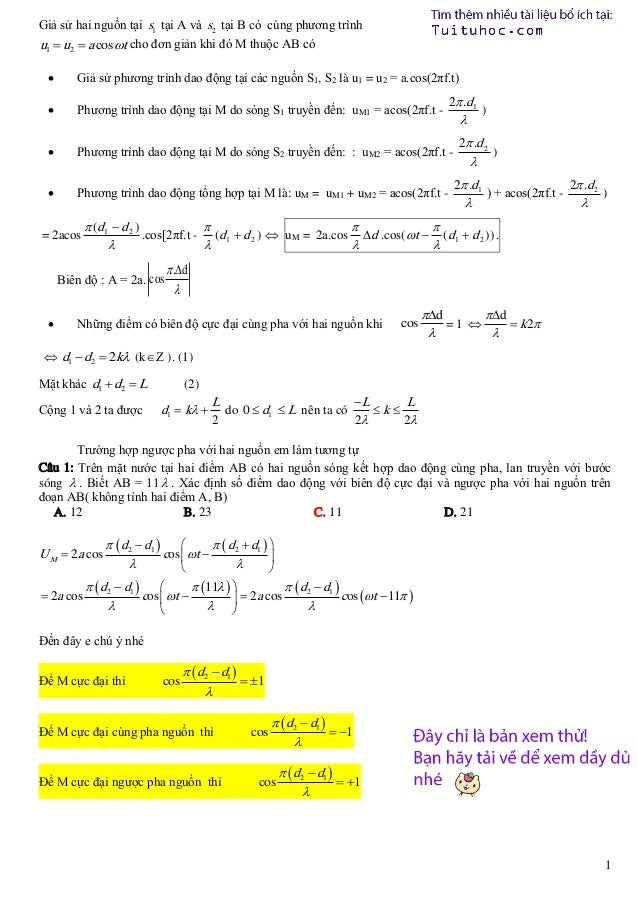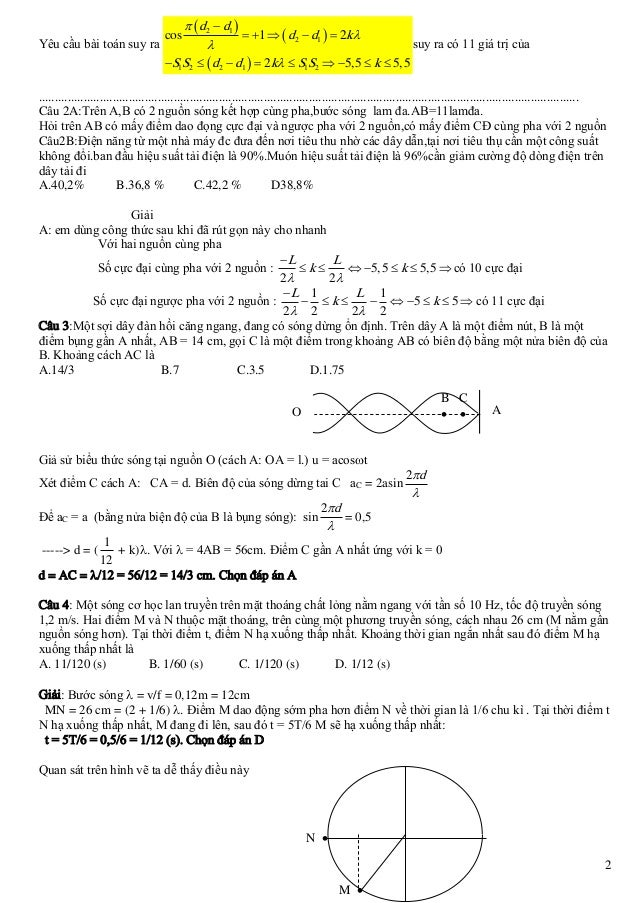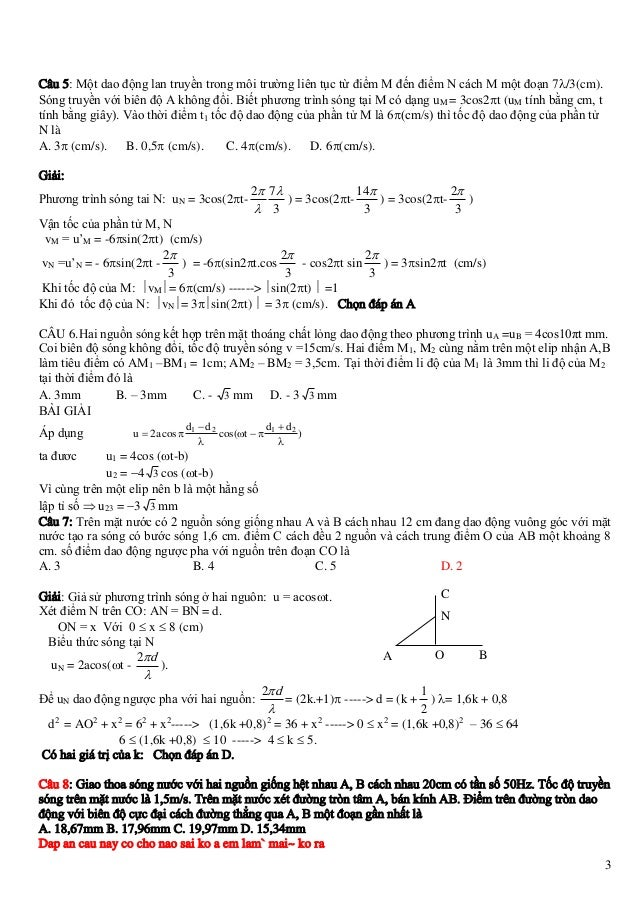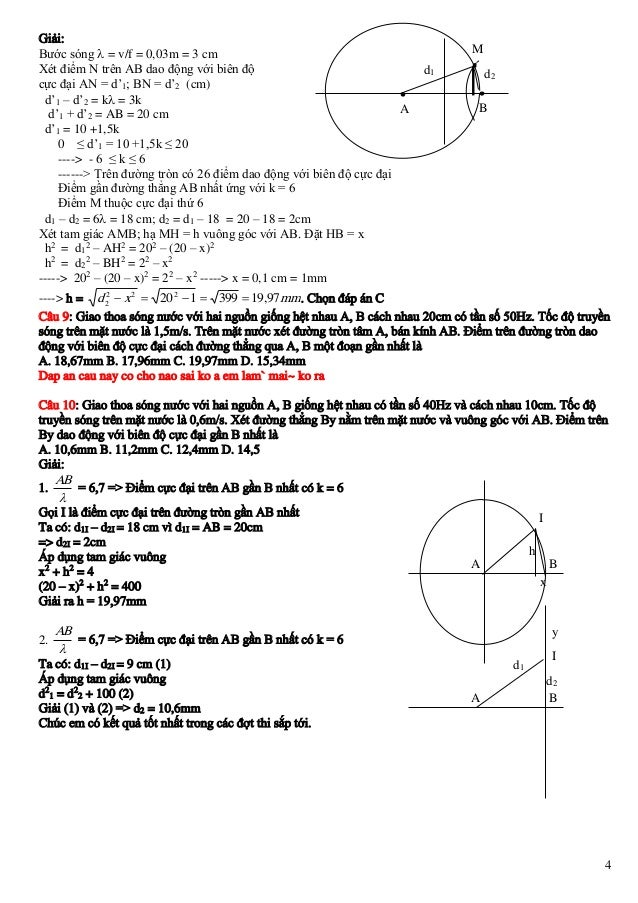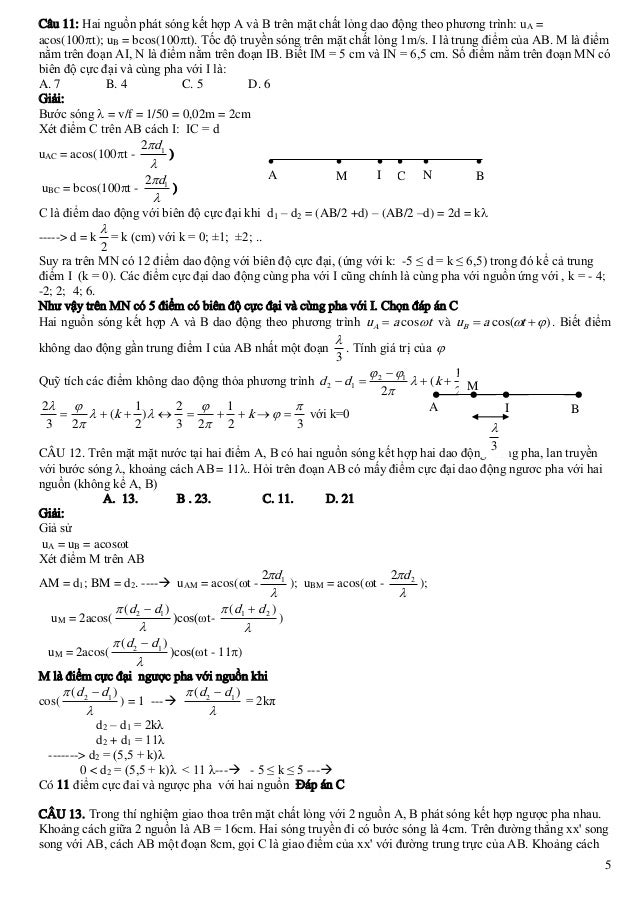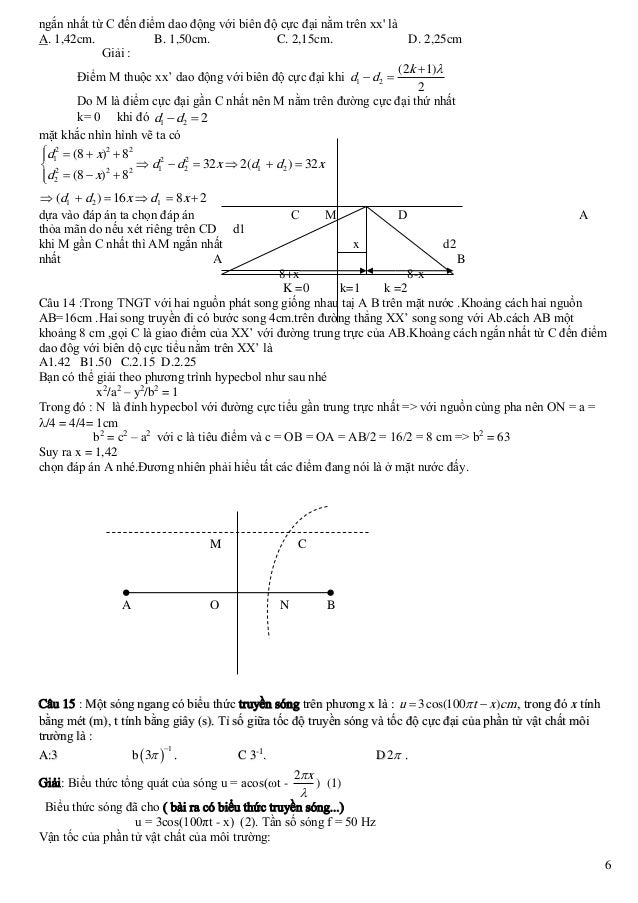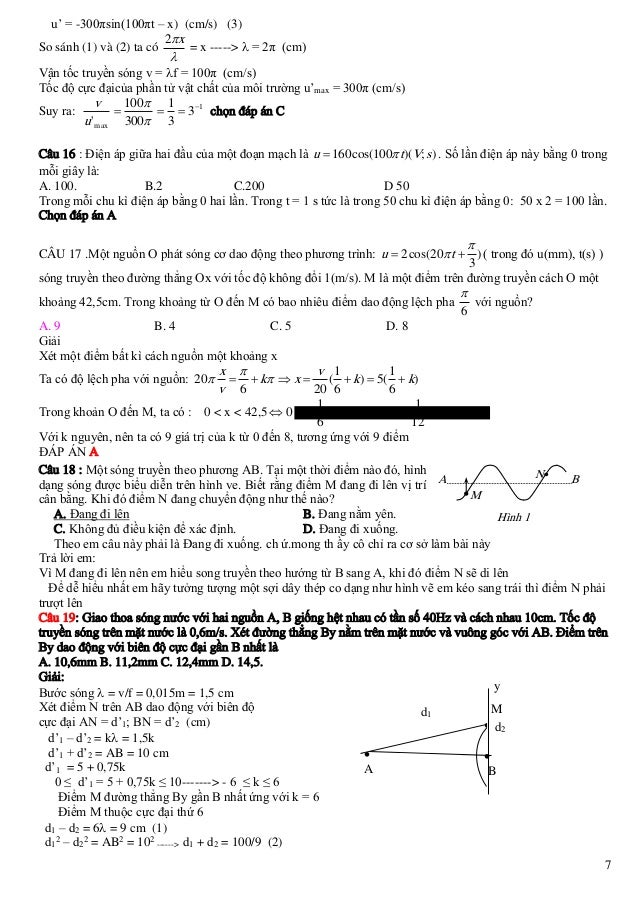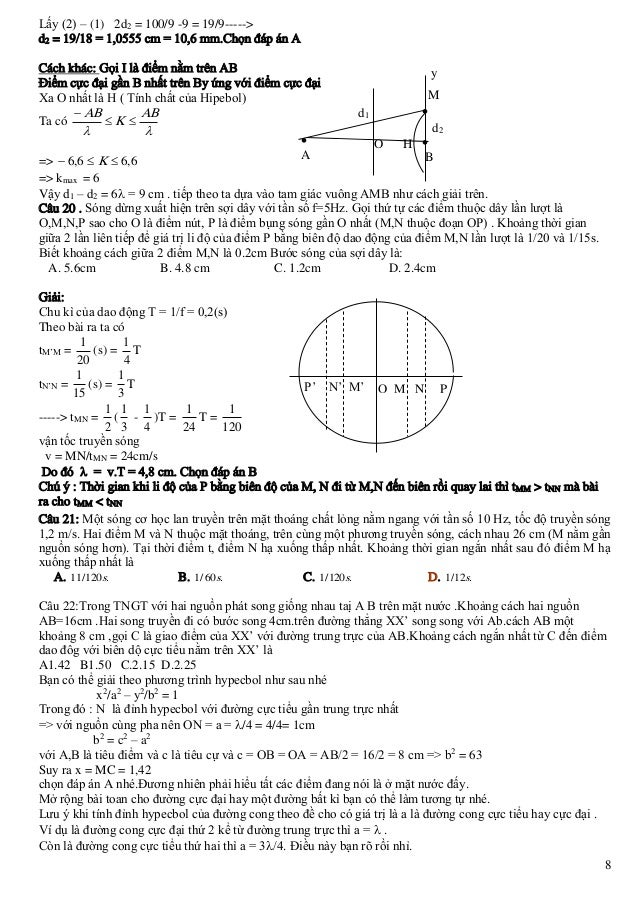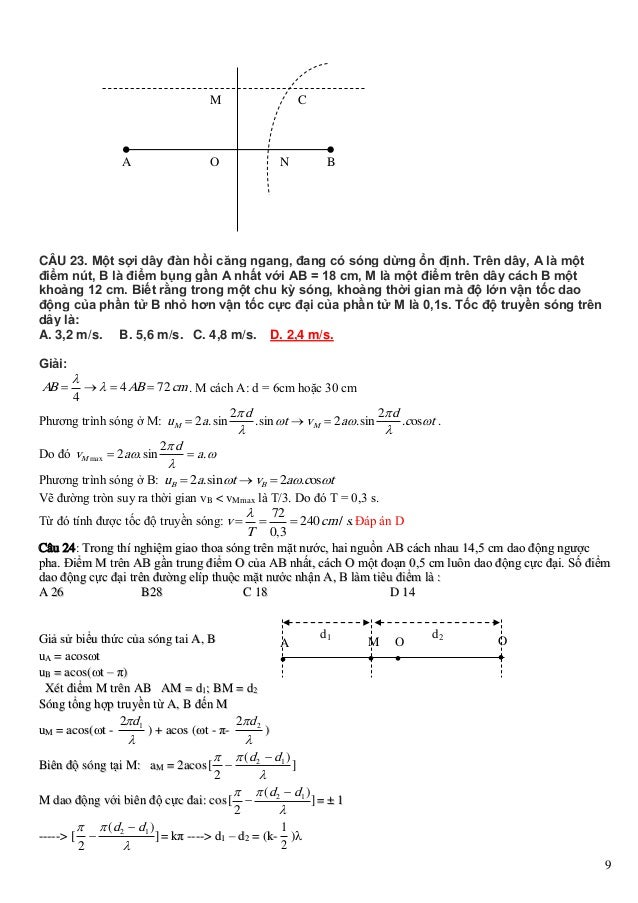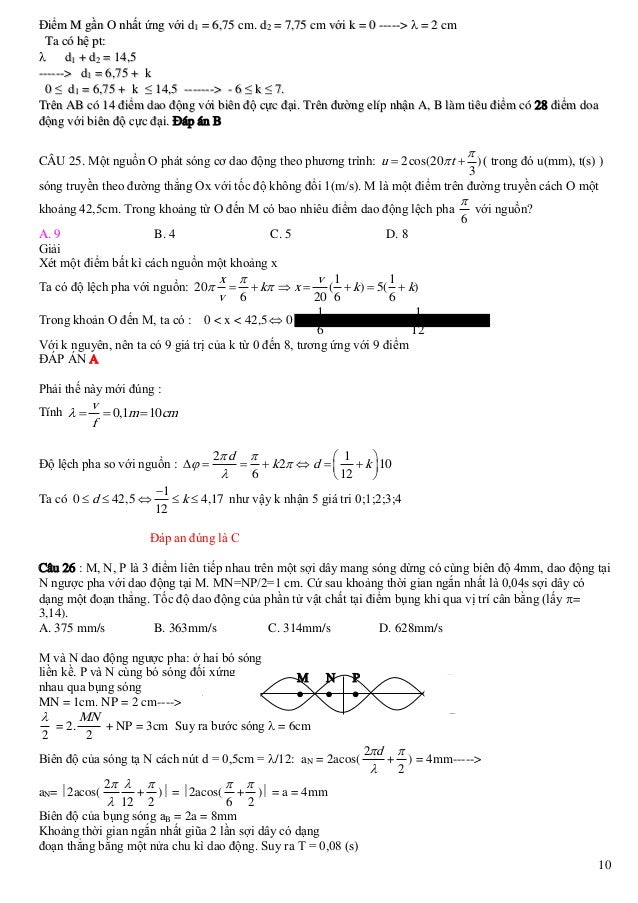Upcoming SlideShare
×
• Full Name
Comment goes here.

Are you sure you want to Yes No• Be the first to comment

### Giải chi tiết một số câu sóng cơ

1. 1. Giả sử hai nguồn tại s1 tại A và s2 tại B có cùng phương trình u1  u2  a cos t cho đơn giản khi đó M thuộc AB có  Giả sử phương trình dao động tại các nguồn S1, S2 là u1 = u2 = a.cos(2πf.t)  Phương trình dao động tại M do sóng S1 truyền đến: uM1 = acos(2πf.t -  Phương trình dao động tại M do sóng S2 truyền đến: : uM2 = acos(2πf.t -  Phương trình dao động tổng hợp tại M là: uM = uM1 + uM2 = acos(2πf.t - = 2acos  ) 2 .d 2  2 .d1  ) ) + acos(2πf.t - 2 .d 2  )  ( d1  d 2 )    .cos[2πf.t - (d1  d 2 )  uM = 2a.cos d .cos( t  (d1  d 2 )) .     Biên độ : A = 2a. cos  2 .d1  .d  Những điểm có biên độ cực đại cùng pha với hai nguồn khi cos d d  k 2 =1     d1  d2  2k (k  Ζ ). (1) Mặt khác d1  d2  L Cộng 1 và 2 ta được (2) d1  k  L 2 do 0  d1  L nên ta có L L k 2 2 Trường hợp ngược pha với hai nguồn em làm tương tự Câu 1: Trên mặt nước tại hai điểm AB có hai nguồn sóng kết hợp dao động cùng pha, lan truyền với bước sóng  . Biết AB = 11  . Xác định số điểm dao động với biên độ cực đại và ngược pha với hai nguồn trên đoạn AB( không tính hai điểm A, B) A. 12 B. 23 C. 11 D. 21 U M  2a cos  2a cos   d2  d1    d2  d1    cos  t         d2  d1   11     d 2  d1   cos  t  cos t  11    2a cos      Đến đây e chú ý nhé Để M cực đại thì cos   d2  d1   1  Để M cực đại cùng pha nguồn thì Để M cực đại ngược pha nguồn thì cos   d2  d1   1  cos   d2  d1   1  1
2. 2.   d2  d1   1   d2  d1   2k Yêu cầu bài toán suy ra suy ra có 11 giá trị của  S1S2   d 2  d1   2k  S1S2  5,5  k  5,5 cos ........................................................................................................................................................................ Câu 2A:Trên A,B có 2 nguồn sóng kết hợp cùng pha,bước sóng lam đa.AB=11lamđa. Hỏi trên AB có mấy điểm dao đọng cực đại và ngược pha với 2 nguồn,có mấy điểm CĐ cùng pha với 2 nguồn Câu2B:Điện năng từ một nhà máy đc đưa đến nơi tiêu thu nhờ các dây dẫn,tại nơi tiêu thụ cần một công suất không đổi.ban đầu hiệu suất tải điện là 90%.Muón hiệu suất tải điện là 96%cần giảm cường độ dòng điện trên dây tải đi A.40,2% B.36,8 % C.42,2 % D38,8% Giải A: em dùng công thức sau khi đã rút gọn này cho nhanh Với hai nguồn cùng pha L L k  5,5  k  5,5  có 10 cực đại Số cực đại cùng pha với 2 nguồn : 2 2 L 1 L 1  k   5  k  5  có 11 cực đại Số cực đại ngược pha với 2 nguồn : 2 2 2 2 Câu 3:Một sợi dây đàn hồi căng ngang, đang có sóng dừng ổn định. Trên dây A là một điểm nút, B là một điểm bụng gần A nhất, AB = 14 cm, gọi C là một điểm trong khoảng AB có biên độ bằng một nửa biên độ của B. Khoảng cách AC là A.14/3 B.7 C.3.5 D.1.75 B C   O Giả sử biểu thức sóng tại nguồn O (cách A: OA = l.) u = acost Xét điểm C cách A: CA = d. Biên độ của sóng dừng tai C aC = 2asin Để aC = a (bằng nửa biện độ của B là bụng sóng): sin 2d  A 2d  = 0,5 1 + k). Với  = 4AB = 56cm. Điểm C gần A nhất ứng với k = 0 12 d = AC = /12 = 56/12 = 14/3 cm. Chọn đáp án A -----> d = ( Câu 4: Một sóng cơ học lan truyền trên mặt thoáng chất lỏng nằm ngang với tần số 10 Hz, tốc độ truyền sóng 1,2 m/s. Hai điểm M và N thuộc mặt thoáng, trên cùng một phương truyền sóng, cách nhau 26 cm (M nằm gần nguồn sóng hơn). Tại thời điểm t, điểm N hạ xuống thấp nhất. Khoảng thời gian ngắn nhất sau đó điểm M hạ xuống thấp nhất là A. 11/120 (s) B. 1/60 (s) C. 1/120 (s) D. 1/12 (s) Giải: Bước sóng  = v/f = 0,12m = 12cm MN = 26 cm = (2 + 1/6) . Điểm M dao động sớm pha hơn điểm N về thời gian là 1/6 chu kì . Tại thời điểm t N hạ xuống thấp nhất, M đang đi lên, sau đó t = 5T/6 M sẽ hạ xuống thấp nhất: t = 5T/6 = 0,5/6 = 1/12 (s). Chọn đáp án D Quan sát trên hình vẽ ta dễ thấy điều này N  2 M 
3. 3. Câu 5: Một dao động lan truyền trong môi trường liên tục từ điểm M đến điểm N cách M một đoạn 7/3(cm). Sóng truyền với biên độ A không đổi. Biết phương trình sóng tại M có dạng uM = 3cos2t (uM tính bằng cm, t tính bằng giây). Vào thời điểm t1 tốc độ dao động của phần tử M là 6(cm/s) thì tốc độ dao động của phần tử N là A. 3 (cm/s). B. 0,5 (cm/s). C. 4(cm/s). D. 6(cm/s). Giải: Phương trình sóng tai N: uN = 3cos(2t- 2 7 14 2 ) = 3cos(2t) = 3cos(2t)  3 3 3 Vận tốc của phần tử M, N vM = u’M = -6sin(2t) (cm/s) 2 2 2 vN =u’N = - 6sin(2t ) = -6(sin2t.cos - cos2t sin ) = 3sin2t (cm/s) 3 3 3 Khi tốc độ của M: vM= 6(cm/s) ------> sin(2t)  =1 Khi đó tốc độ của N: vN= 3sin(2t)  = 3 (cm/s). Chọn đáp án A CÂU 6.Hai nguồn sóng kết hợp trên mặt thoáng chất lỏng dao động theo phương trình uA =uB = 4cos10πt mm. Coi biên độ sóng không đổi, tốc độ truyền sóng v =15cm/s. Hai điểm M1, M2 cùng nằm trên một elip nhận A,B làm tiêu điểm có AM1 –BM1 = 1cm; AM2 – BM2 = 3,5cm. Tại thời điểm li độ của M1 là 3mm thì li độ của M2 tại thời điểm đó là A. 3mm B. – 3mm C. - 3 mm D. - 3 3 mm BÀI GIẢI Áp dụng u  2a cos  d1  d 2 d  d2 cos(t   1 )   ta đươc u1 = 4cos (t-b) u2 = 4 3 cos (t-b) Vì cùng trên một elip nên b là một hằng số lập tỉ số  u23 = 3 3 mm Câu 7: Trên mặt nước có 2 nguồn sóng giống nhau A và B cách nhau 12 cm đang dao động vuông góc với mặt nước tạo ra sóng có bước sóng 1,6 cm. điểm C cách đều 2 nguồn và cách trung điểm O của AB một khoảng 8 cm. số điểm dao động ngược pha với nguồn trên đoạn CO là A. 3 B. 4 C. 5 D. 2 Giải: Giả sử phương trình sóng ở hai nguôn: u = acost. Xét điểm N trên CO: AN = BN = d. ON = x Với 0  x  8 (cm) Biểu thức sóng tại N 2d uN = 2acos(t ).  2d C N A O B 1 ) = 1,6k + 0,8  2 d2 = AO2 + x2 = 62 + x2-----> (1,6k +0,8)2 = 36 + x2 -----> 0  x2 = (1,6k +0,8)2 – 36  64 6  (1,6k +0,8)  10 -----> 4  k  5. Có hai giá trị của k: Chọn đáp án D. Để uN dao động ngược pha với hai nguồn: = (2k.+1) -----> d = (k + Câu 8: Giao thoa sóng nước với hai nguồn giống hệt nhau A, B cách nhau 20cm có tần số 50Hz. Tốc độ truyền sóng trên mặt nước là 1,5m/s. Trên mặt nước xét đường tròn tâm A, bán kính AB. Điểm trên đường tròn dao động với biên độ cực đại cách đường thẳng qua A, B một đoạn gần nhất là A. 18,67mm B. 17,96mm C. 19,97mm D. 15,34mm Dap an cau nay co cho nao sai ko a em lam` mai~ ko ra 3
4. 4. Giải: Bước sóng  = v/f = 0,03m = 3 cm Xét điểm N trên AB dao động với biên độ cực đại AN = d’1; BN = d’2 (cm)  d’1 – d’2 = k = 3k A d’1 + d’2 = AB = 20 cm d’1 = 10 +1,5k 0 ≤ d’1 = 10 +1,5k ≤ 20 ----> - 6 ≤ k ≤ 6 ------> Trên đường tròn có 26 điểm dao động với biên độ cực đại Điểm gần đường thẳng AB nhất ứng với k = 6 Điểm M thuộc cực đại thứ 6 d1 – d2 = 6 = 18 cm; d2 = d1 – 18 = 20 – 18 = 2cm Xét tam giác AMB; hạ MH = h vuông góc với AB. Đặt HB = x h2 = d12 – AH2 = 202 – (20 – x)2 h2 = d22 – BH2 = 22 – x2 -----> 202 – (20 – x)2 = 22 – x2 -----> x = 0,1 cm = 1mm d1 M  d2  B 2 ----> h = d 2  x2  20 2  1  399  19,97mm . Chọn đáp án C Câu 9: Giao thoa sóng nước với hai nguồn giống hệt nhau A, B cách nhau 20cm có tần số 50Hz. Tốc độ truyền sóng trên mặt nước là 1,5m/s. Trên mặt nước xét đường tròn tâm A, bán kính AB. Điểm trên đường tròn dao động với biên độ cực đại cách đường thẳng qua A, B một đoạn gần nhất là A. 18,67mm B. 17,96mm C. 19,97mm D. 15,34mm Dap an cau nay co cho nao sai ko a em lam` mai~ ko ra Câu 10: Giao thoa sóng nước với hai nguồn A, B giống hệt nhau có tần số 40Hz và cách nhau 10cm. Tốc độ truyền sóng trên mặt nước là 0,6m/s. Xét đường thẳng By nằm trên mặt nước và vuông góc với AB. Điểm trên By dao động với biên độ cực đại gần B nhất là A. 10,6mm B. 11,2mm C. 12,4mm D. 14,5 Giải: 1. AB = 6,7 => Điểm cực đại trên AB gần B nhất có k = 6  Gọi I là điểm cực đại trên đường tròn gần AB nhất Ta có: d1I – d2I = 18 cm vì d1I = AB = 20cm => d2I = 2cm Áp dụng tam giác vuông x2 + h2 = 4 (20 – x)2 + h2 = 400 Giải ra h = 19,97mm 2. I h A B x AB = 6,7 => Điểm cực đại trên AB gần B nhất có k = 6  Ta có: d1I – d2I = 9 cm (1) Áp dụng tam giác vuông d21 = d22 + 100 (2) Giải (1) và (2) => d2 = 10,6mm Chúc em có kết quả tốt nhất trong các đợt thi sắp tới. y d1 A I d2 B 4
5. 5. Câu 11: Hai nguồn phát sóng kết hợp A và B trên mặt chất lỏng dao động theo phương trình: uA = acos(100t); uB = bcos(100t). Tốc độ truyền sóng trên mặt chất lỏng 1m/s. I là trung điểm của AB. M là điểm nằm trên đoạn AI, N là điểm nằm trên đoạn IB. Biết IM = 5 cm và IN = 6,5 cm. Số điểm nằm trên đoạn MN có biên độ cực đại và cùng pha với I là: A. 7 B. 4 C. 5 D. 6 Giải: Bước sóng  = v/f = 1/50 = 0,02m = 2cm Xét điểm C trên AB cách I: IC = d 2d1 uAC = acos(100t )        A I C N M B 2d1 uBC = bcos(100t )  C là điểm dao động với biên độ cực đại khi d1 – d2 = (AB/2 +d) – (AB/2 –d) = 2d = k -----> d = k  = k (cm) với k = 0; ±1; ±2; .. 2 Suy ra trên MN có 12 điểm dao động với biên độ cực đại, (ứng với k: -5 ≤ d = k ≤ 6,5) trong đó kể cả trung điểm I (k = 0). Các điểm cực đại dao động cùng pha với I cũng chính là cùng pha với nguồn ứng với , k = - 4; -2; 2; 4; 6. Như vậy trên MN có 5 điểm có biên độ cực đại và cùng pha với I. Chọn đáp án C Hai nguồn sóng kết hợp A và B dao động theo phương trình u A  a cost và uB  a cos(t   ) . Biết điểm  . Tính giá trị của  3   1 1   (k  )M  Quỹ tích các điểm không dao động thỏa phương trình d 2  d1  2 2 2 2  1 2  1  A I    ( k  )     k    với k=0 3 2 2 3 2 2 3  không dao động gần trung điểm I của AB nhất một đoạn B 3 CÂU 12. Trên mặt mặt nước tại hai điểm A, B có hai nguồn sóng kết hợp hai dao động cùng pha, lan truyền với bước sóng , khoảng cách AB = 11. Hỏi trên đoạn AB có mấy điểm cực đại dao động ngươc pha với hai nguồn (không kể A, B) A. 13. B . 23. C. 11. D. 21 Giải: Giả sử uA = uB = acost Xét điểm M trên AB 2d1 2d 2 AM = d1; BM = d2. ---- uAM = acos(t ); uBM = acos(t );   ( d 2  d1 )  (d1  d 2 ) )cos(ωt)    ( d 2  d1 ) uM = 2acos( )cos(ωt - 11)   uM = 2acos( M là điểm cực đại ngược pha với nguồn khi  ( d 2  d1 )  ( d 2  d1 ) cos( ) = 1 --- = 2kπ   d2 – d1 = 2kλ d2 + d1 = 11λ -------> d2 = (5,5 + k)λ 0 < d2 = (5,5 + k)λ < 11 λ--- - 5 ≤ k ≤ 5 --- Có 11 điểm cực đai và ngược pha với hai nguồn Đáp án C CÂU 13. Trong thí nghiệm giao thoa trên mặt chất lỏng với 2 nguồn A, B phát sóng kết hợp ngược pha nhau. Khoảng cách giữa 2 nguồn là AB = 16cm. Hai sóng truyền đi có bước sóng là 4cm. Trên đường thẳng xx' song song với AB, cách AB một đoạn 8cm, gọi C là giao điểm của xx' với đường trung trực của AB. Khoảng cách 5
6. 6. ngắn nhất từ C đến điểm dao động với biên độ cực đại nằm trên xx' là A. 1,42cm. B. 1,50cm. C. 2,15cm. D. 2,25cm Giải : (2k  1) Điểm M thuộc xx’ dao động với biên độ cực đại khi d1  d 2  2 Do M là điểm cực đại gần C nhất nên M nằm trên đường cực đại thứ nhất k= 0 khi đó d1  d2  2 mặt khắc nhìn hình vẽ ta có d12  (8  x)2  82  2  d12  d2  32 x  2(d1  d2 )  32 x  2 2 2 d2  (8  x)  8   (d1  d2 )  16 x  d1  8x  2 dựa vào đáp án ta chọn đáp án thỏa mãn do nếu xét riêng trên CD d1 khi M gần C nhất thì AM ngắn nhất nhất A C M D x A d2 B 8+x 8-x K =0 k=1 k =2 Câu 14 :Trong TNGT với hai nguồn phát song giống nhau taị A B trên mặt nước .Khoảng cách hai nguồn AB=16cm .Hai song truyền đi có bước song 4cm.trên đường thẳng XX’ song song với Ab.cách AB một khoảng 8 cm ,gọi C là giao điểm của XX’ với đường trung trực của AB.Khoảng cách ngắn nhất từ C đến điểm dao đôg với biên dộ cực tiểu nằm trên XX’ là A1.42 B1.50 C.2.15 D.2.25 Bạn có thể giải theo phương trình hypecbol như sau nhé x2/a2 – y2/b2 = 1 Trong đó : N là đỉnh hypecbol với đường cực tiểu gần trung trực nhất => với nguồn cùng pha nên ON = a = /4 = 4/4= 1cm b2 = c2 – a2 với c là tiêu điểm và c = OB = OA = AB/2 = 16/2 = 8 cm => b2 = 63 Suy ra x = 1,42 chọn đáp án A nhé.Đương nhiên phải hiểu tất các điểm đang nói là ở mặt nước đấy. M A O C N B Câu 15 : Một sóng ngang có biểu thức truyền sóng trên phương x là : u  3cos(100 t  x)cm , trong đó x tính bằng mét (m), t tính bằng giây (s). Tỉ số giữa tốc độ truyền sóng và tốc độ cực đại của phần tử vật chất môi trường là : 1 A:3 b  3  . C 3-1. D 2 . 2x Giải: Biểu thức tổng quát của sóng u = acos(t ) (1)  Biểu thức sóng đã cho ( bài ra có biểu thức truyền sóng...) u = 3cos(100πt - x) (2). Tần số sóng f = 50 Hz Vận tốc của phần tử vật chất của môi trường: 6
7. 7. u’ = -300πsin(100πt – x) (cm/s) (3) 2x So sánh (1) và (2) ta có = x ----->  = 2π (cm)  Vận tốc truyền sóng v = f = 100π (cm/s) Tốc độ cực đạicủa phần tử vật chất của môi trường u’max = 300π (cm/s) v 100 1 Suy ra:    31 chọn đáp án C u' max 300 3 Câu 16 : Điện áp giữa hai đầu của một đoạn mạch là u  160cos(100 t )(V; s) . Số lần điện áp này bằng 0 trong mỗi giây là: A. 100. B.2 C.200 D 50 Trong mỗi chu kì điện áp bằng 0 hai lần. Trong t = 1 s tức là trong 50 chu kì điện áp bằng 0: 50 x 2 = 100 lần. Chọn đáp án A  CÂU 17 .Một nguồn O phát sóng cơ dao động theo phương trình: u  2cos(20 t  ) ( trong đó u(mm), t(s) ) 3 sóng truyền theo đường thẳng Ox với tốc độ không đổi 1(m/s). M là một điểm trên đường truyền cách O một khoảng 42,5cm. Trong khoảng từ O đến M có bao nhiêu điểm dao động lệch pha A. 9 B. 4 C. 5 Giải Xét một điểm bất kì cách nguồn một khoảng x Ta có độ lệch pha với nguồn: 20  với nguồn? 6 D. 8 x  v 1 1   k  x  (  k)  5(  k) v 6 20 6 6 1 1 k 8,333 0 < x < 42,5  0 5(  k) 42,5   6 12 Với k nguyên, nên ta có 9 giá trị của k từ 0 đến 8, tương ứng với 9 điểm ĐÁP ÁN A Câu 18 : Một sóng truyền theo phương AB. Tại một thời điểm nào đó, hình N B dạng sóng được biểu diễn trên hình ve. Biết rằng điểm M đang đi lên vị trí A M cân bằng. Khi đó điểm N đang chuyển động như thế nào? A. Đang đi lên B. Đang nằm yên. Hình 1 C. Không đủ điều kiện để xác định. D. Đang đi xuống. Theo em câu này phải là Đang đi xuống. ch ứ.mong th ầy cô chỉ ra cơ sở làm bài này Trả lời em: Vì M đang đi lên nên em hiểu song truyền theo hướng từ B sang A, khi đó điểm N sẽ di lên Để dễ hiểu nhất em hãy tưởng tượng một sợi dây thép co dạng như hình vẽ em kéo sang trái thì điểm N phải trượt lên Câu 19: Giao thoa sóng nước với hai nguồn A, B giống hệt nhau có tần số 40Hz và cách nhau 10cm. Tốc độ truyền sóng trên mặt nước là 0,6m/s. Xét đường thẳng By nằm trên mặt nước và vuông góc với AB. Điểm trên By dao động với biên độ cực đại gần B nhất là A. 10,6mm B. 11,2mm C. 12,4mm D. 14,5. Giải: y Bước sóng  = v/f = 0,015m = 1,5 cm Xét điểm N trên AB dao động với biên độ M d1 cực đại AN = d’1; BN = d’2 (cm)  d2 d’1 – d’2 = k = 1,5k d’1 + d’2 = AB = 10 cm   d’1 = 5 + 0,75k A B 0 ≤ d’1 = 5 + 0,75k ≤ 10-------> - 6 ≤ k ≤ 6 Điểm M đường thẳng By gần B nhất ứng với k = 6 Điểm M thuộc cực đại thứ 6 d1 – d2 = 6 = 9 cm (1) d12 – d22 = AB2 = 102 ------> d1 + d2 = 100/9 (2) Trong khoản O đến M, ta có : 7
8. 8. Lấy (2) – (1) 2d2 = 100/9 -9 = 19/9-----> d2 = 19/18 = 1,0555 cm = 10,6 mm.Chọn đáp án A Cách khác: Gọi I là điểm nằm trên AB Điểm cực đại gần B nhất trên By ứng với điểm cực đại Xa O nhất là H ( Tính chất của Hipebol)  AB AB K Ta có    A =>  6,6  K  6,6 y M  d1 O d2  H B => kmax = 6 Vậy d1 – d2 = 6 = 9 cm . tiếp theo ta dựa vào tam giác vuông AMB như cách giải trên. Câu 20 . Sóng dừng xuất hiện trên sợi dây với tần số f=5Hz. Gọi thứ tự các điểm thuộc dây lần lượt là O,M,N,P sao cho O là điểm nút, P là điểm bụng sóng gần O nhất (M,N thuộc đoạn OP) . Khoảng thời gian giữa 2 lần liên tiếp để giá trị li độ của điểm P bằng biên độ dao động của điểm M,N lần lượt là 1/20 và 1/15s. Biết khoảng cách giữa 2 điểm M,N là 0.2cm Bước sóng của sợi dây là: A. 5.6cm B. 4.8 cm C. 1.2cm D. 2.4cm Giải: Chu kì của dao động T = 1/f = 0,2(s) Theo bài ra ta có 1 1 tM’M = (s) = T 20 4 1 1 tN’N = (s) = T P’ N’ M’ O M N P 15 3 1 1 1 1 1 -----> tMN = ( - )T = T= 2 3 4 24 120 vận tốc truyền sóng v = MN/tMN = 24cm/s Do đó  = v.T = 4,8 cm. Chọn đáp án B Chú ý : Thời gian khi li độ của P bằng biên độ của M, N đi từ M,N đến biên rồi quay lai thì tMM > tNN mà bài ra cho tMM < tNN Câu 21: Một sóng cơ học lan truyền trên mặt thoáng chất lỏng nằm ngang với tần số 10 Hz, tốc độ truyền sóng 1,2 m/s. Hai điểm M và N thuộc mặt thoáng, trên cùng một phương truyền sóng, cách nhau 26 cm (M nằm gần nguồn sóng hơn). Tại thời điểm t, điểm N hạ xuống thấp nhất. Khoảng thời gian ngắn nhất sau đó điểm M hạ xuống thấp nhất là A. 11/120s. B. 1/ 60s. C. 1/120s. D. 1/12s. Câu 22:Trong TNGT với hai nguồn phát song giống nhau taị A B trên mặt nước .Khoảng cách hai nguồn AB=16cm .Hai song truyền đi có bước song 4cm.trên đường thẳng XX’ song song với Ab.cách AB một khoảng 8 cm ,gọi C là giao điểm của XX’ với đường trung trực của AB.Khoảng cách ngắn nhất từ C đến điểm dao đôg với biên dộ cực tiểu nằm trên XX’ là A1.42 B1.50 C.2.15 D.2.25 Bạn có thể giải theo phương trình hypecbol như sau nhé x2/a2 – y2/b2 = 1 Trong đó : N là đỉnh hypecbol với đường cực tiểu gần trung trực nhất => với nguồn cùng pha nên ON = a = /4 = 4/4= 1cm b2 = c2 – a2 với A,B là tiêu điểm và c là tiêu cự và c = OB = OA = AB/2 = 16/2 = 8 cm => b2 = 63 Suy ra x = MC = 1,42 chọn đáp án A nhé.Đương nhiên phải hiểu tất các điểm đang nói là ở mặt nước đấy. Mở rộng bài toan cho đường cực đại hay một đường bất kì bạn có thể làm tương tự nhé. Lưu ý khi tính đỉnh hypecbol của đường cong theo đề cho có giá trị là a là đường cong cực tiểu hay cực đại . Ví dụ là đường cong cực đại thứ 2 kể từ đường trung trực thì a =  . Còn là đường cong cực tiểu thứ hai thì a = 3/4. Điều này bạn rõ rồi nhỉ. 8
9. 9. M A C O N B CÂU 23. Một sợi dây đàn hồi căng ngang, đang có sóng dừng ổn định. Trên dây, A là một điểm nút, B là điểm bụng gần A nhất với AB = 18 cm, M là một điểm trên dây cách B một khoảng 12 cm. Biết rằng trong một chu kỳ sóng, khoảng thời gian mà độ lớn vận tốc dao động của phần tử B nhỏ hơn vận tốc cực đại của phần tử M là 0,1s. Tốc độ truyền sóng trên dây là: A. 3,2 m/s. B. 5,6 m/s. C. 4,8 m/s. D. 2,4 m/s. Giải: AB   4    4 AB  72 cm . M cách A: d = 6cm hoặc 30 cm Phương trình sóng ở M: uM  2a .sin Do đó vM max  2a.sin 2 d 2 d  .sin t  vM  2a.sin 2 d  .cost .  a.  Phương trình sóng ở B: uB  2a.sin t  vB  2a.cost Vẽ đường tròn suy ra thời gian vB < vMmax là T/3. Do đó T = 0,3 s.  72 Từ đó tính được tốc độ truyền sóng: v    240 cm / s. Đáp án D T 0,3 Câu 24: Trong thí nghiệm giao thoa sóng trên mặt nước, hai nguồn AB cách nhau 14,5 cm dao động ngược cách pha. Điểm M trên AB gần trung điểm O của AB nhất, cách O một đoạn 0,5 cm luôn dao động cực đại. Số điểm dao động cực đại trên đường elíp thuộc mặt nước nhận A, B làm tiêu điểm là : A 26 B28 C 18 D 14 Giả sử biểu thức của sóng tai A, B uA = acost uB = acos(t – π) Xét điểm M trên AB AM = d1; BM = d2 Sóng tổng hợp truyền từ A, B đến M 2d1 2d 2 uM = acos(t ) + acos (t - π) A A   d1 M  O  d2 O      ( d 2  d1 ) Biên độ sóng tại M: aM = 2acos [  ] 2    ( d 2  d1 ) M dao động với biên độ cực đai: cos [  ]= ± 1 2  1   ( d 2  d1 ) ] = kπ ----> d1 – d2 = (k- ) -----> [  2 2  9
10. 10. Điểm M gần O nhất ứng với d1 = 6,75 cm. d2 = 7,75 cm với k = 0 ----->  = 2 cm Ta có hệ pt:  d1 + d2 = 14,5 ------> d1 = 6,75 + k 0 ≤ d1 = 6,75 + k ≤ 14,5 -------> - 6 ≤ k ≤ 7. Trên AB có 14 điểm dao động với biên độ cực đại. Trên đường elíp nhận A, B làm tiêu điểm có 28 điểm doa động với biên độ cực đại. Đáp án B  CÂU 25. Một nguồn O phát sóng cơ dao động theo phương trình: u  2cos(20 t  ) ( trong đó u(mm), t(s) ) 3 sóng truyền theo đường thẳng Ox với tốc độ không đổi 1(m/s). M là một điểm trên đường truyền cách O một khoảng 42,5cm. Trong khoảng từ O đến M có bao nhiêu điểm dao động lệch pha A. 9 B. 4 C. 5 Giải Xét một điểm bất kì cách nguồn một khoảng x Ta có độ lệch pha với nguồn: 20  với nguồn? 6 D. 8 x  v 1 1   k  x  (  k)  5(  k) v 6 20 6 6 1 1 0 < x < 42,5  0 5(  k) 42,5   6 12 Với k nguyên, nên ta có 9 giá trị của k từ 0 đến 8, tương ứng với 9 điểm ĐÁP ÁN A Trong khoản O đến M, ta có : k 8,333 Phải thế này mới đúng : Tính   v  0,1m  10cm f Độ lệch pha so với nguồn :   Ta có 0  d  42,5  2 d    1   k 2  d    k 10 6  12  1  k  4,17 như vậy k nhận 5 giá tri 0;1;2;3;4 12 Đáp an đúng là C Câu 26 : M, N, P là 3 điểm liên tiếp nhau trên một sợi dây mang sóng dừng có cùng biên độ 4mm, dao động tại N ngược pha với dao động tại M. MN=NP/2=1 cm. Cứ sau khoảng thời gian ngắn nhất là 0,04s sợi dây có dạng một đoạn thẳng. Tốc độ dao động của phần tử vật chất tại điểm bụng khi qua vị trí cân bằng (lấy = 3,14). A. 375 mm/s B. 363mm/s C. 314mm/s D. 628mm/s M và N dao động ngược pha: ở hai bó sóng liền kề. P và N cùng bó sóng đối xứng nhau qua bụng sóng MN = 1cm. NP = 2 cm----> M  N  P   MN = 2. + NP = 3cm Suy ra bước sóng  = 6cm 2 2 Biên độ của sóng tạ N cách nút d = 0,5cm = /12: aN = 2acos( 2     aN= 2acos( + ) = 2acos( + ) = a = 4mm  12 2 6 2 Biên độ của bụng sóng aB = 2a = 8mm Khoảng thời gian ngắn nhất giũa 2 lần sợi dây có dạng đoạn thẳng bằng một nửa chu kì dao động. Suy ra T = 0,08 (s) 2d  +  ) = 4mm-----> 2 10

### Be the first to comment

•#### VinhDuong7

Apr. 28, 2018
•#### ThanhThanh273

May. 10, 2018
•#### phmlan8

May. 15, 2018
•#### ThoaPham22

Jun. 7, 2018
•#### NguynThHoa2

Jul. 8, 2018
•#### NgNgcTunThnh

Aug. 18, 2018
•#### JeonSomi1

Aug. 27, 2018
•#### LazyCat3

Apr. 4, 2019
•#### HNgc43

Apr. 24, 2019
•#### HngNhung73

Jul. 20, 2019
•#### VietThanh10

Sep. 18, 2019
•#### DuynM56

Sep. 26, 2019
•#### ThanhHuynV1

Oct. 10, 2019
•#### nguyenma3

Oct. 11, 2019
•#### AnMinh19

Nov. 12, 2019
•#### Nganhi1809

Dec. 12, 2019
•#### VnTho22

Mar. 5, 2020
•#### TrnThng68

Nov. 2, 2020
•#### TrnThng68

Nov. 2, 2020
•#### HHong111

Feb. 28, 2021

Total views

165,852

On Slideshare

0

From embeds

0

Number of embeds

2,342

1,035

Shares

0